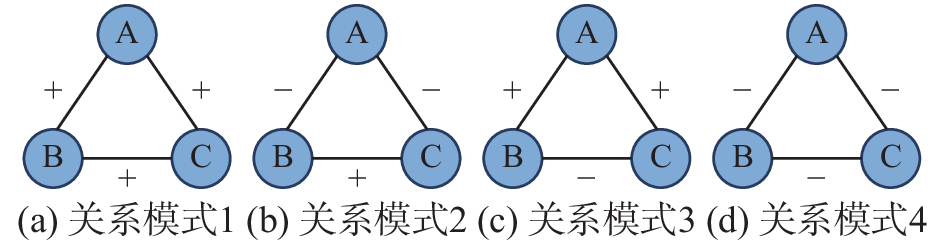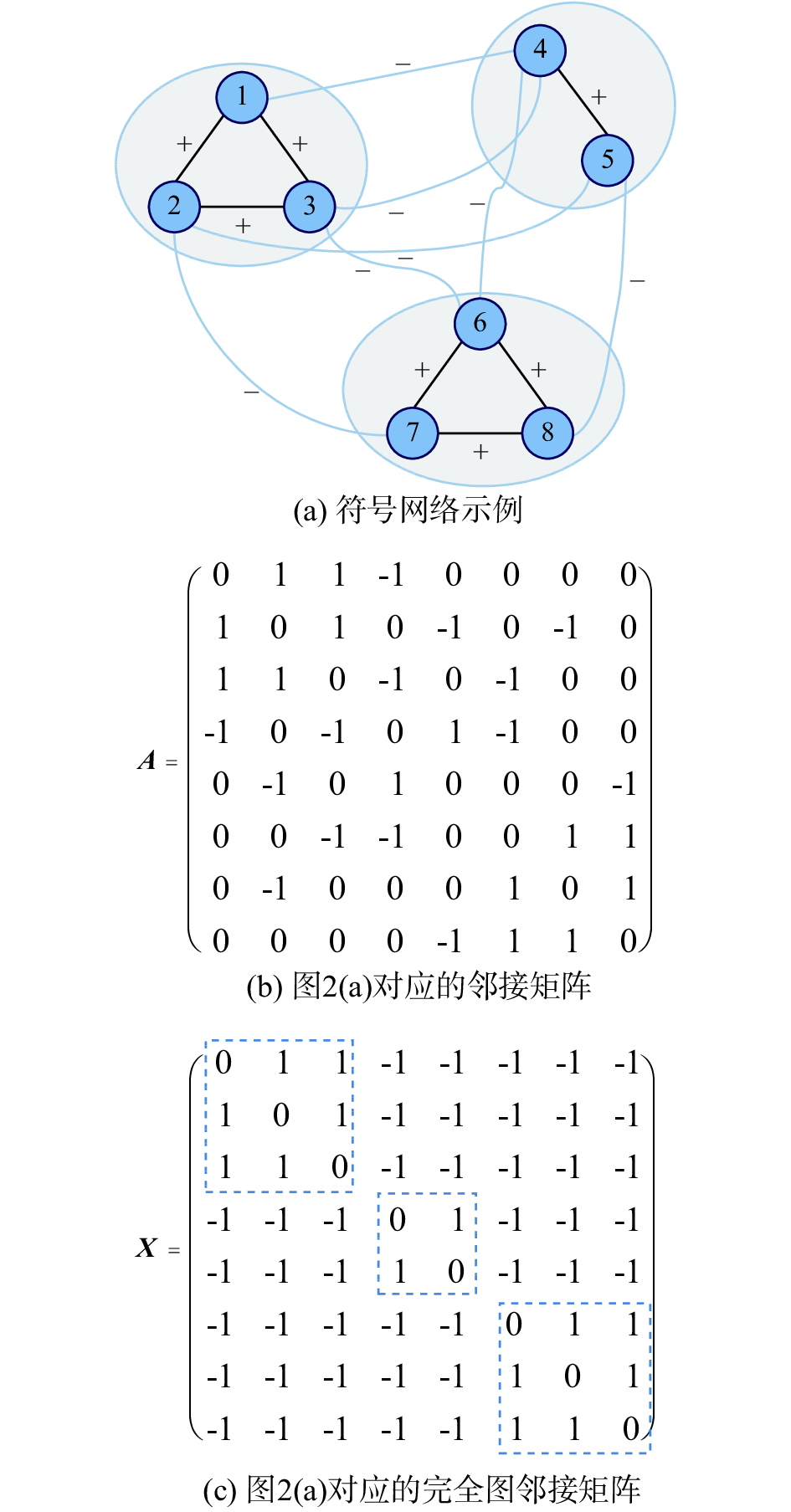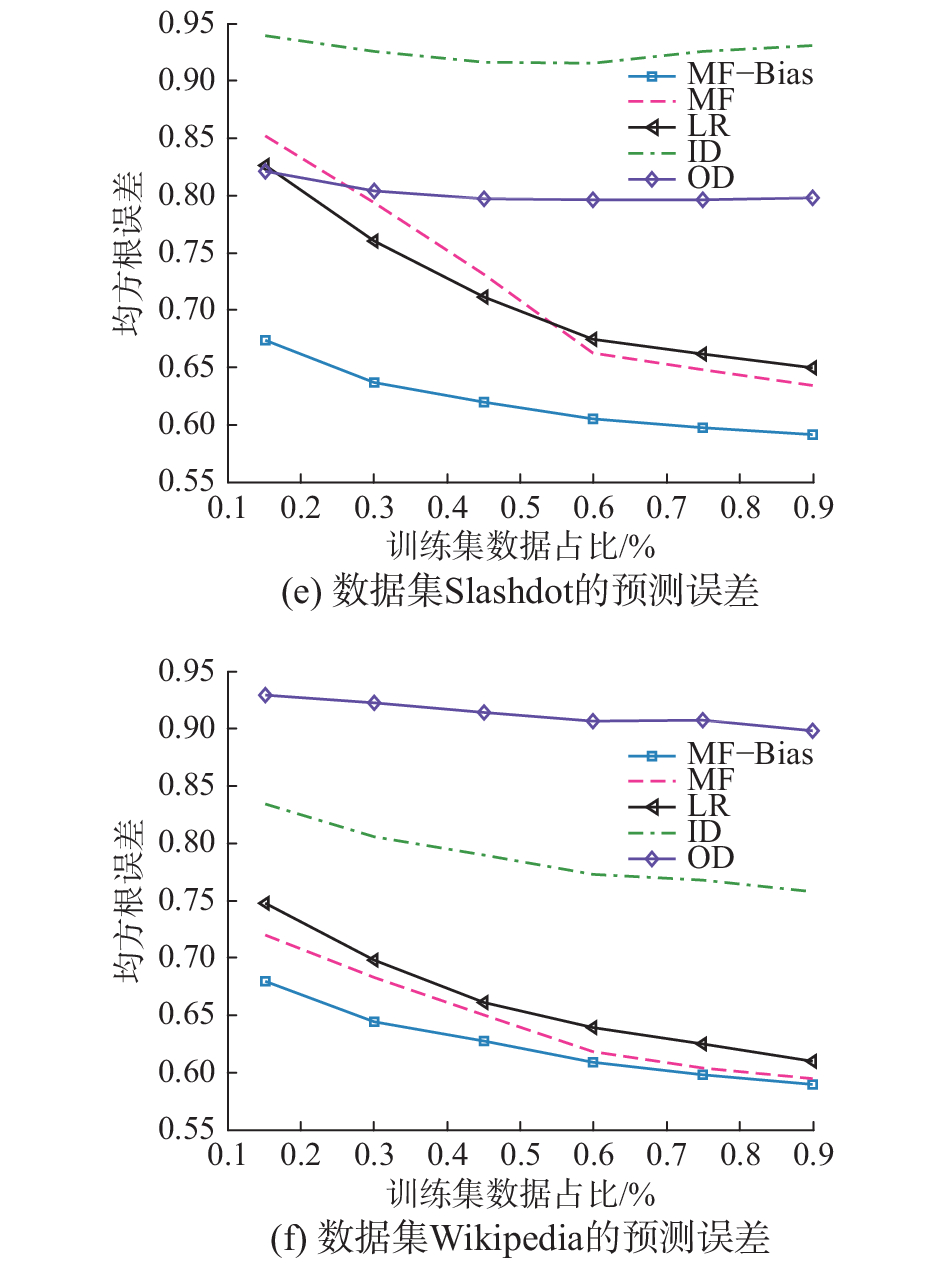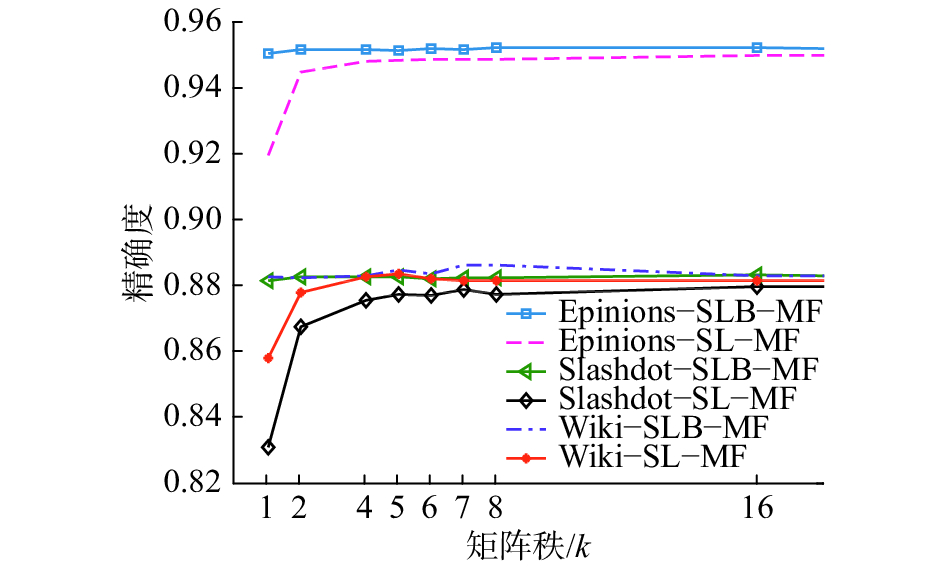﻿ 符号网络的局部标注特征与预测方法
«上一篇文章快速检索 高级检索

 智能系统学报2018, Vol. 13Issue (3): 437-444  DOI: 10.11992/tis.2017100270

引用本文SU Xiaoping, SONG Yurong. Local labeling features and a prediction method for a signed network[J]. CAAI Transactions on Intelligent Systems, 2018, 13(3), 437-444. DOI: 10.11992/tis.201710027.文章历史

1. 南京工业职业技术学院 计算机与软件学院，江苏 南京 210046;
2. 南京邮电大学 自动化学院，江苏 南京 210003

Local labeling features and a prediction method for a signed network
SU Xiaoping1, SONG Yurong2
1. School of Computer and Software Engineering, Nanjing Institute of Industry Technology, Nanjing 210046, China;
2. College of Automation, Nanjing University of Posts and Telecommunications, Nanjing 210003, China
Abstract: A complex network may be considered as a symbol network when links have a positive or negative sign attribute. In signed social networks, positive links represent a trust (friends) relationship between users. In contrast, negative links indicate distrust (hostility). An important task in a signed network is to define a signed network based on partial observation to predict an unknown symbol. Through analysis, we found that for a signed network with weak structural balance, its adjacent matrix has a global low-rank characteristic and the prediction of the link sign can be approximated as a low-rank matrix factorization. However, in a basic low-rank model, it is difficult to sufficiently utilize the local behavior features for labeling the signs of links between the neighboring nodes. Herein, a low-rank matrix factorization model with bias was proposed. In this model, the sign features of the exit and entry links of a neighboring node were introduced to improve the precision of sign prediction. Experiments based on real data revealed that the low-rank model with bias can obtain better prediction results than other benchmark algorithms and that the proposed algorithm performed with a high efficiency.
Key words: signed networks    sign prediction    low rank    matrix factorization    signed bias    structural balance theory    weak structural balance theory    status theory

1 相关理论 1.1 基本定义

 ${A_{ij}} = \left\{ \begin{array}{l}1,\quad i{\text{与}}j{\text{之间有正边}}\\0,\quad {\text{边符号未知或不存在边}}\\ - 1,\quad i{\text{与}}j{\text{之间有负边}}\end{array} \right.$

1.2 结构平衡与弱结构平衡理论Download: 图 1 符号网中4种三角形关系模式 Fig. 1 Four relationship patterns of triads in signed network

1.3 矩阵的秩与结构平衡Download: 图 2 弱平衡结构与矩阵的低秩特性 Fig. 2 Weakly balanced network and low-rank structure

 $\begin{array}{l}\begin{array}{*{20}{c}}{\min } & {{\rm{Rank}}({{X}})}\end{array}\\\begin{array}{*{20}{c}}{{\rm{s}}{\rm{.t}}{\rm{.}}} & \begin{array}{l}{X_{ij}} = {A_{ij}},\forall e(i,j) \in O,\\{X_{ij}} \in \{ \pm 1\} ,\forall e(i,j) \notin O\end{array}\end{array}\end{array}$ (1)

2 符号预测

 $\begin{array}{*{20}{c}}\!\!\!\!\!\!\!\! {\mathop {\min }\limits_{{{{P}}^{\rm{T}}},{{Q}} \in {\mathbb{R}^{k \times n}}} }{C = \displaystyle\sum\limits_{e(i,j) \in {\rm O}} {l({A_{ij}},\sum\limits_{k = 1}^\kappa {{P_{ik}}{Q_{kj}}} )} + \lambda {{\left\| {{P}} \right\|}^2} + \lambda {{\left\| {{Q}} \right\|}^2}} \end{array}$ (2)

 ${b_{ij}} = \mu + U{i_{\rm{out}}} + U{j_{\rm{in}}}$ (3)Download: 图 3 标注行为的偏置现象 Fig. 3 Bias behavior of signed edges

${b_{ij}}$ 的值能够很好地反映待预测边两端节点的局部标注行为和行为偏好，将标注偏好反映在预测的目标函数，得到较基本模型更为精细的预测模型：

 $\begin{array}{c}\mathop {\min }\limits_{{{{P}}^{\rm{T}}},{{Q}} \in {{\mathbb{R}}^{k \times n}}} C = \displaystyle\sum\limits_{e(i,j) \in {\rm O}} {l({A_{ij}} - ({b_{ij}} + \sum\limits_{k = 1}^\kappa {{P_{ik}}{Q_{kj}})} } ) + \\\;\;\;\;\;\;\;\;\lambda (\left\| {{P}} \right\|_1^2 + \left\| {{Q}} \right\|_1^2 + U{i_{\rm{out}}}^2 + U{j_{\rm{in}}}^2)\end{array}$ (4)

 $\begin{array}{c}\mathop {\min }\limits_{{{{P}}^{\rm{T}}},{{Q}} \in {{\mathbb{R}}^{k \times n}}}C = {\displaystyle\sum\limits_{e(i,j) \in {\rm O}} {\left\| {{A_{ij}} - ({b_{ij}} + \sum\limits_{k = 1}^\kappa {{P_{ik}}{Q_{kj}})} } \right\|} ^2} + \\\;\;\;\;\;\;\;\;\;\;\;\;\;\lambda (\left\| {{P}} \right\|_1^2 + \left\| {{Q}} \right\|_1^2 + U{i_{\rm{out}}}^2 + U{j_{\rm{in}}}^2)\end{array}$ (5)

 ${P_i} \leftarrow {P_i} + \alpha ({e_{ij}}{Q_j} - \lambda {P_i})$ (6)
 ${Q_j} \leftarrow {Q_j} + \alpha ({e_{ij}}{P_i} - \lambda {Q_j})$ (7)
 $U{i_{\rm{out}}} \leftarrow U{i_{\rm{out}}} + \alpha ({e_{ij}} - \lambda U{i_{\rm{out}}})$ (8)
 $U{j_{\rm{in}}} \leftarrow U{j_{\rm{in}}} + \alpha ({e_{ij}} - \lambda U{j_{\rm{in}}})$ (9)

3 实验结果与分析 3.1 数据集描述表 1 数据集统计特征 Tab.1 Statistics of datasets

3.2 预测效果与分析

1) OutDegree(简写为OD)：若 $d_{{\rm{out}}}^{\rm{ + }}(i) \geqslant d_{{\rm{out}}}^ -$ ，则被预测边 $e(i,j)$ 的符号为正，反之为负。

2) InDegree(简写为ID)：若 $d_{{\rm{in}}}^{\rm{ + }}(j) \geqslant d_{{\rm{in}}}^ - (j)$ ，则被预测边 $e(i,j)$ 的符号为正，反之为负。

3) LR (logistic regression)：将符号预测问题看作二值分类问题，采用逻辑回归模型训练分类器，得到了较高的预测精度。

4) MF(matrix factorization)：由Hsieh等提出的基本矩阵分解模型。

3.2.1 评价指标

1)均方根误差(RMSE)

 ${\rm{RMSE}} = \sqrt {\frac{{\displaystyle\sum\limits_{i = 1}^n {{{({p_i} - {a_i})}^2}} }}{n}}$ (10)

2)精确性(Accuracy)

 ${\rm{Accuracy}} = \frac{{{\rm{TP}} + {\rm{TN}}}}{{P + N}}$ (11)Download: 图 4 3个符号网络的预测结果 Fig. 4 Three signed networks predicted results
3.2.2 实验参数设置

3.2.3 实验结果及讨论

3.3 秩与预测精度Download: 图 5 预测精度与矩阵的秩 $\kappa$ 间的关系 Fig. 5 The relation between prediction accuracy and $\kappa$
4 结束语

  程苏琦, 沈华伟, 张国清,等. 符号网络研究综述[J]. 软件学报, 2014, 25(1): 1-15. CHENG Suqi, SHEN Huawei, ZHANG Guoqing et al. Survey of signed network research[J]. Journal of software, 2014, 25(1): 1-15. (0)  TANG Jiliang, AGGARWAL C, LIU Huan. Recommendations in signed social networks[C]//Proceedings of the 25th International Conference on World Wide Web. Montréal, Canada, 2016: 31–40. (0)  LI Dong, XU Zhiming, CHAKRABORTY N, et al. Polarity related influence maximization in signed social networks[J]. PLoS one, 2014, 9(7): e102199. DOI:10.1371/journal.pone.0102199 (0)  EVERETT M G, BORGATTI S P. Networks containing negative ties[J]. Social networks, 2014, 38: 111-120. DOI:10.1016/j.socnet.2014.03.005 (0)  HEIDER F. Attitudes and cognitive organization[J]. The Journal of psychology: interdisciplinary and applied, 1946, 21(1): 107-112. DOI:10.1080/00223980.1946.9917275 (0)  SZELL M, LAMBIOTTE R, THURNER S. Multirelational organization of large-scale social networks in an online world[J]. Proceedings of the national academy of sciences of the United States of America, 2010, 107(31): 13636-13641. DOI:10.1073/pnas.1004008107 (0)  CHU Lingyang, WANG Zhefeng, PEI Jian, et al. Finding gangs in war from signed networks[C]//Proceedings of the 22nd ACM SIGKDD International Conference on Knowledge Discovery and Data Mining. San Francisco, USA, 2016: 1505–1514. (0)  DAVIS J A. Clustering and structural balance in graphs[J]. Human relations, 1967, 20(2): 181-187. DOI:10.1177/001872676702000206 (0)  LESKOVEC J, HUTTENLOCHER D, KLEINBERG J. Signed networks in social media[C]//Proceedings of the SIGCHI Conference on Human Factors in Computing Systems. Atlanta, USA, 2010: 1361–1370. (0)  GUHA R, KUMAR R, RAGHAVAN P, et al. Propagation of trust and distrust[C]//Proceedings of the 13th International Conference on World Wide Web. New York, USA, 2004: 403–412. (0)  LESKOVEC J, HUTTENLOCHER D, KLEINBERG J. Predicting positive and negative links in online social networks[C]//Proceedings of the 19th International Conference on World Wide Web. Raleigh, USA, 2010: 641–650. (0)  WANG Guannan, GAO Hui, CHEN Lian, et al. Predicting positive and negative relationships in large social networks[J]. PLoS one, 2015, 10(6): e0129530. DOI:10.1371/journal.pone.0129530 (0)  CHIANG K Y, NATARAJAN N, TEWARI A, et al. Exploiting longer cycles for link prediction in signed networks[C]//Proceedings of the 20th ACM international Conference on Information and Knowledge Management. Glasgow, Scotland, UK, 2011: 1157–1162. (0)  蓝梦微, 李翠平, 王绍卿, 等. 符号社会网络中正负关系预测算法研究综述[J]. 计算机研究与发展, 2015, 52(2): 410-422. LAN Mengwei, LI Cuiping, Wang Shaoqing, et al. Survey of sign prediction algorithms in signed social networks[J]. Journal of computer research and development, 2015, 52(2): 410-422. DOI:10.7544/issn1000-1239.2015.20140210 (0)  SONG Dongjin, MEYER D A. Link sign prediction and ranking in signed directed social networks[J]. Social network analysis and mining, 2015, 5: 52. DOI:10.1007/s13278-015-0288-7 (0)  KUNEGIS J, SCHMIDT S, LOMMATZSCH A, et al. Spectral analysis of signed graphs for clustering, prediction and visualization[C]//Proceedings of the 2010 SIAM International Conference on Data Mining. Columbus, USA, 2010: 559–570. (0)  HSIEH C J, CHIANG K Y, DHILLON I S. Low rank modeling of signed networks[C]//Proceedings of the 18th ACM SIGKDD International Conference on Knowledge Discovery and Data Mining. Beijing, China, 2012: 507–515. (0)  MENON A K, ELKAN C. Link prediction via matrix factorization[C]//Proceedings of the 2011 European Conference on Machine Learning and Knowledge Discovery in Databases. Athens, Greece, 2011: 437–452. (0)  AGRAWAL P, GARG V K, NARAYANAM R. Link label prediction in signed social networks[C]//Proceedings of the 23rd International Joint Conference on Artificial Intelligence. Beijing, China, 2013: 2591–2597. (0)  CARTWRIGHT D, HARARY F. Structural balance: a generalization of Heider’s theory[J]. Psychological review, 1956, 63(5): 277-293. DOI:10.1037/h0046049 (0)  CANDÈS E J, TAO T. The power of convex relaxation: near-optimal matrix completion[J]. IEEE transactions on information theory, 2010, 56(5): 2053-2080. DOI:10.1109/TIT.2010.2044061 (0)